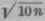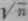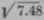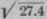If the given number contains but three (or less) significant figures, the square or cube is found in the column headed n2 or n3, opposite the given number in the column headed n. If the given number contains more than three significant figures, proceed in a manner similar to that described for extracting roots. To square a number, place the decimal point between the first and second significant figures and find in the column headedortwo consecutive numbers, one of which shall be a little greater and the other a little less than the given number. The remainder of the work is exactly as heretofore described. To locate the decimal point, employ the principle that the square of any number contains either twice as many figures as the number squared or twice as many less one. If the column headedis used, the square will contain twice as many figures, while if the column headedis used, the square will contain twice as many figures as the number squared, less one. If the number contains an integral part, the principle is applied to the integral part only; if the number is wholly decimal, there will be twice as many ciphers following the decimal in the square or twice as many plus one as in the number squared, depending on whetherorcolumn is used. For example. will contain five figures in the integral part; 4516.22 will contain eight figures in the integral part, all after the fifth being denoted by ciplers: .00294538 will have five ciphers following the decimal point: .0524362 will have two ciphers following the decimal point.

### Example

(a) 273.422 =? (6) .0524362 =?

### Solution

(a) Placing the decimal point between the first and second significant figures, the result is 2.7342; this number occurs between 2.73313 =and 2.73496 =in the column headedThe first difference is 2.73496 - 2.73313

= 183; the second difference is 2.73420 - 2.73313 = 107; and 107/183 = .584+, or .58. Hence, 273.422 - 74,758, correct to five significant figures.

(6) Shifting the decimal point to between the first and second significant figures, we get the number 5.2436, which falls between 5.23450 =and 5.24404 =The first difference is 954; the second difference is 910; 910/954 = .953+, or .95. Hence, .0524362 = .0027495, to five significant figures.

A number is cubed in exactly the same manner. using the column headedoraccording to whether the first period of the significant part of the number contains one, two, or three figures, respectively. If the number contains an integral part, the number of figures in the integral part of the cube will be three times as many as in the given number if column headedis used; it will be three times as many less 1 if the column headedused; and it will be three times as many less 2 if the column headedis used. If the given number is wholly decimal the cube will have either three times, three times plus one, or three times plus two, as many ciphers following the decimal as there are ciphers following the decimal point in the given number.

### Example

(a) 129.6843=? (6) .764423 =? (c) .0324253 =?

### Solution

(a) Placing the decimal point between the first and second significant figures, the number 1.29684 is found between 1.29664 =and 1.29862 =The first difference is 198; the second difference is 20; and 20/198 = .101+, or .10. Hence, the first five significant figures are 21810; the number of figures in the integral part of the cube is 3X3 - 2=7; and 129.6843 = 2,181,000, correct to five significant figures.

(b) 7.64420 occurs between 7.64032 =and 7.64603 =The first difference is 571; the second difference is 388; and 388/571 = .679+, or .68. Hence, the first five significant figures are 44668; the number of ciphers following the decimal point is 3 X 0 = 0; and .764423 = .44668, correct to five significant figures.

(c) 3.2425 falls between 3.24278 =and 3.23961 =The first difference is 317; the second difference is 289; 289/317 = .911+, or .91. Hence, the first five significant figures are 34091; the number of ciphers following the decimal point is 3 X 1 + 1 = 4; and .0324253 = .000034091, correct to five significant figures.